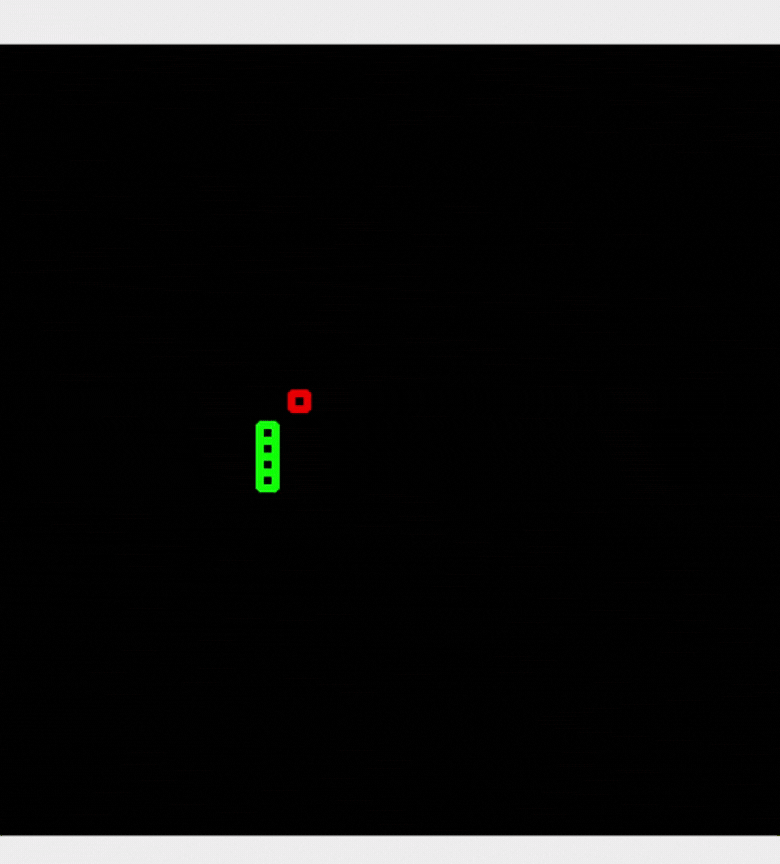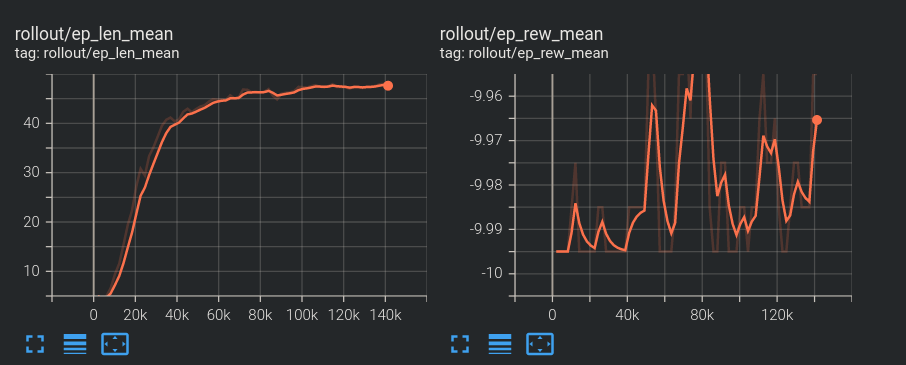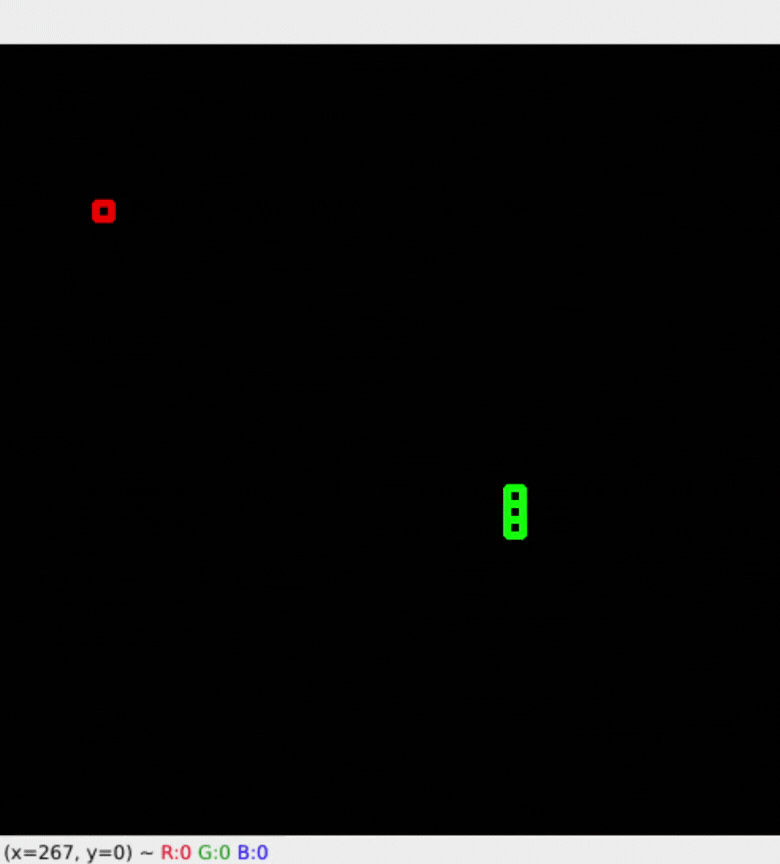## Reinforcement Learning in Python with Stable Baselines 3

#### Using Custom Environments

My guess is that most people are going to want to use reinforcement learning on their own environments, rather than just Open AI's gym environments. While conceptually, all you have to do is convert some environment to a gym environment, this process can actually turn out to be fairly tricky and I would argue that the hardest part to reinforcement learning is actually in the engineering of your environment's observations and rewards for the agent.

So, first, let's get ourselves some environment to use. Games tend to make good environments, so I think a Snake game could be quite fitting. I searched around for a nice short/simple Snake game, and I found: https://github.com/TheAILearner/Snake-Game-using-OpenCV-Python/blob/master/snake_game_using_opencv.ipynb

I took the notebook and converted it to a script here:

```# source: https://github.com/TheAILearner/Snake-Game-using-OpenCV-Python/blob/master/snake_game_using_opencv.ipynb
import numpy as np
import cv2
import random
import time

def collision_with_apple(apple_position, score):
apple_position = [random.randrange(1,50)*10,random.randrange(1,50)*10]
score += 1
return apple_position, score

return 1
else:
return 0

def collision_with_self(snake_position):
return 1
else:
return 0

img = np.zeros((500,500,3),dtype='uint8')
# Initial Snake and Apple position
snake_position = [[250,250],[240,250],[230,250]]
apple_position = [random.randrange(1,50)*10,random.randrange(1,50)*10]
score = 0
prev_button_direction = 1
button_direction = 1
while True:
cv2.imshow('a',img)
cv2.waitKey(1)
img = np.zeros((500,500,3),dtype='uint8')
# Display Apple
cv2.rectangle(img,(apple_position,apple_position),(apple_position+10,apple_position+10),(0,0,255),3)
# Display Snake
for position in snake_position:
cv2.rectangle(img,(position,position),(position+10,position+10),(0,255,0),3)

# Takes step after fixed time
t_end = time.time() + 0.05
k = -1
while time.time() < t_end:
if k == -1:
k = cv2.waitKey(1)
else:
continue

# 0-Left, 1-Right, 3-Up, 2-Down, q-Break
# a-Left, d-Right, w-Up, s-Down

if k == ord('a') and prev_button_direction != 1:
button_direction = 0
elif k == ord('d') and prev_button_direction != 0:
button_direction = 1
elif k == ord('w') and prev_button_direction != 2:
button_direction = 3
elif k == ord('s') and prev_button_direction != 3:
button_direction = 2
elif k == ord('q'):
break
else:
button_direction = button_direction
prev_button_direction = button_direction

# Change the head position based on the button direction
if button_direction == 1:
elif button_direction == 0:
elif button_direction == 2:
elif button_direction == 3:

# Increase Snake length on eating apple
apple_position, score = collision_with_apple(apple_position, score)

else:
snake_position.pop()

# On collision kill the snake and print the score
if collision_with_boundaries(snake_head) == 1 or collision_with_self(snake_position) == 1:
font = cv2.FONT_HERSHEY_SIMPLEX
img = np.zeros((500,500,3),dtype='uint8')
cv2.putText(img,'Your Score is {}'.format(score),(140,250), font, 1,(255,255,255),2,cv2.LINE_AA)
cv2.imshow('a',img)
cv2.waitKey(0)
break

cv2.destroyAllWindows()```

The main changes made are around the snippet:

```	t_end = time.time() + 0.2
k = -1
while time.time() < t_end:
if k == -1:
k = cv2.waitKey(125)
```

Changing 0.2 to more like 0.05 and the waitKey to 1. We want to step as quickly as possible here.Playing this, it's a simple snake game where you attempt to get the apple without running into yourself or going out of bounds. To convert this to a gym environment, we need to follow the following structure:

```import gym
from gym import spaces

class CustomEnv(gym.Env):
"""Custom Environment that follows gym interface"""

def __init__(self, arg1, arg2, ...):
super(CustomEnv, self).__init__()
# Define action and observation space
# They must be gym.spaces objects
# Example when using discrete actions:
self.action_space = spaces.Discrete(N_DISCRETE_ACTIONS)
# Example for using image as input (channel-first; channel-last also works):
self.observation_space = spaces.Box(low=0, high=255,
shape=(N_CHANNELS, HEIGHT, WIDTH), dtype=np.uint8)

def step(self, action):
...
return observation, reward, done, info
def reset(self):
...
return observation  # reward, done, info can't be included
def render(self, mode='human'):
...
def close (self):
...```

So, first, we need to consider what would go where. In the initialization method, we probably would start off by just defining our action space and observation space. What actions can we take? We can see these actions in the following snippet of the snake code:

```if button_direction == 1:
elif button_direction == 0:
elif button_direction == 2:
elif button_direction == 3:

This tells us that there are 4 clear possible actions, so this means we have a discrete action space of 4, so our first bit of code in the init method will be:

`self.action_space = spaces.Discrete(4)`

Next, we need our observation. In the case of games, it can be tempting to just pass the image of the game, but this can often be very challenging for a reinforcement learning algorithm to learn, and it can often be far better to engineer your own, more specific, and hopefully more useful, observations. In the game of snake, for example, what might matter?

We need to know where the snake's head is, where the apple is, in relation to the head, and where the rest of the snake's body is. I highly encourage you to maybe come up with your own observations, feel free to play and tinker here. The only slightly challenging part is, every time you eat an apple, the length of the snake is increased by 1. We need our observation to be a fixed size, whether the snake is 3 units long, or 300. My propsal for an observation will be:

`observation = [head_x, head_y, apple_delta_x, apple_delta_y, snake_length] + list(self.prev_actions)`

... where `self.prev_actions` needs to be a fixed-size list of previous actions that I expect the agent to be capable of figuring out how to extrapolate to where the rest of the body is based on "snake length." We'll see, and this is exactly what I mean by this being the hard part of reinforcement learning.

...well, the observation and reward!

The reward in this case is fairly obvious I think, we will start with just the snake's size as the reward:

`        self.total_reward = len(self.snake_position) - 3  # start length is 3`

Okay, let's build our gym env. To start, we'll just copy and paste over those functions from the snake game:

```import gym
from gym import spaces

def collision_with_apple(apple_position, score):
apple_position = [random.randrange(1,50)*10,random.randrange(1,50)*10]
score += 1
return apple_position, score

return 1
else:
return 0

def collision_with_self(snake_position):
return 1
else:
return 0```

Next, we need to come up with whatever our length aspiration is. I am sure some of you will come up with ideas for how this could be made more dynamic. For now, I'll just go with: `SNAKE_LEN_GOAL = 30`. As we see how this goes, we can tweak this more later. Let's also bring in our imports:

```import numpy as np
import cv2
import random
import time
from collections import deque```

Next, we'll finish the init method:

```class SnekEnv(gym.Env):

def __init__(self):
super(SnekEnv, self).__init__()
# Define action and observation space
# They must be gym.spaces objects
# Example when using discrete actions:
self.action_space = spaces.Discrete(4)
# Example for using image as input (channel-first; channel-last also works):
self.observation_space = spaces.Box(low=-500, high=500,
shape=(5+SNAKE_LEN_GOAL,), dtype=np.float32)```

Code up to this point:

```import gym
from gym import spaces

SNAKE_LEN_GOAL = 30

def collision_with_apple(apple_position, score):
apple_position = [random.randrange(1,50)*10,random.randrange(1,50)*10]
score += 1
return apple_position, score

return 1
else:
return 0

def collision_with_self(snake_position):
return 1
else:
return 0

class CustomEnv(gym.Env):
"""Custom Environment that follows gym interface"""

def __init__(self, arg1, arg2, ...):
super(CustomEnv, self).__init__()
# Define action and observation space
# They must be gym.spaces objects
# Example when using discrete actions:
self.action_space = spaces.Discrete(N_DISCRETE_ACTIONS)
# Example for using image as input (channel-first; channel-last also works):
self.observation_space = spaces.Box(low=0, high=255,
shape=(N_CHANNELS, HEIGHT, WIDTH), dtype=np.uint8)

def step(self, action):
...
return observation, reward, done, info
def reset(self):
...
return observation  # reward, done, info can't be included
def render(self, mode='human'):
...
def close (self):
...```

I think the next logical method to address will be the reset method, since this is what gets called for every new episode, before we start taking steps. The reset method will essentially set up the start of our environment, as well as returning the first observation for us to start working with. To begin, let's start with the snake game itself:

```
def reset(self):
self.img = np.zeros((500,500,3),dtype='uint8')
# Initial Snake and Apple position
self.snake_position = [[250,250],[240,250],[230,250]]
self.apple_position = [random.randrange(1,50)*10,random.randrange(1,50)*10]
self.score = 0
self.prev_button_direction = 1
self.button_direction = 1

Essentially all we've done here is just copy the code from the snake game and add a bunch of `self.`.

Next, we'll set up reward tracking, as well as build our first observation:

```	self.prev_reward = 0

self.done = False

snake_length = len(self.snake_position)

self.prev_actions = deque(maxlen = SNAKE_LEN_GOAL)  # however long we aspire the snake to be
for i in range(SNAKE_LEN_GOAL):
self.prev_actions.append(-1) # to create history

# create observation:
observation = np.array(observation)

return observation```

That's all for the reset method, no we just need to build the `step` method. Again, it'll be a lot of doing `self.` to previous codes, tracking move histories, and building the observation. The start of the `step` method:

```	def step(self, action):
self.prev_actions.append(action)
cv2.imshow('a',self.img)
cv2.waitKey(1)
self.img = np.zeros((500,500,3),dtype='uint8')
# Display Apple
cv2.rectangle(self.img,(self.apple_position,self.apple_position),(self.apple_position+10,self.apple_position+10),(0,0,255),3)
# Display Snake
for position in self.snake_position:
cv2.rectangle(self.img,(position,position),(position+10,position+10),(0,255,0),3)

# Takes step after fixed time
t_end = time.time() + 0.05
k = -1
while time.time() < t_end:
if k == -1:
k = cv2.waitKey(1)
else:
continue

button_direction = action
# Change the head position based on the button direction
if button_direction == 1:
elif button_direction == 0:
elif button_direction == 2:
elif button_direction == 3:

# Increase Snake length on eating apple
self.apple_position, self.score = collision_with_apple(self.apple_position, self.score)

else:
self.snake_position.pop()

# On collision kill the snake and print the score
if collision_with_boundaries(self.snake_head) == 1 or collision_with_self(self.snake_position) == 1:
font = cv2.FONT_HERSHEY_SIMPLEX
self.img = np.zeros((500,500,3),dtype='uint8')
cv2.putText(self.img,'Your Score is {}'.format(self.score),(140,250), font, 1,(255,255,255),2,cv2.LINE_AA)
cv2.imshow('a',self.img)
self.done = True
```

This is mostly the original snake game code, just turned into OOP. Note the `self.prev_actions.append(action)` for tracking historical actions. Next, we'll track reward delta and make our observation:

```	self.total_reward = len(self.snake_position) - 3  # default length is 3
self.reward = self.total_reward - self.prev_reward
self.prev_reward = self.total_reward

if self.done:
self.reward = -10
info = {}

snake_length = len(self.snake_position)

# create observation:

observation = np.array(observation)

return observation, self.reward, self.done, info
```

Full code is now:

```import gym
from gym import spaces
import numpy as np
import cv2
import random
import time
from collections import deque

SNAKE_LEN_GOAL = 30

def collision_with_apple(apple_position, score):
apple_position = [random.randrange(1,50)*10,random.randrange(1,50)*10]
score += 1
return apple_position, score

return 1
else:
return 0

def collision_with_self(snake_position):
return 1
else:
return 0

class SnekEnv(gym.Env):

def __init__(self):
super(SnekEnv, self).__init__()
# Define action and observation space
# They must be gym.spaces objects
# Example when using discrete actions:
self.action_space = spaces.Discrete(4)
# Example for using image as input (channel-first; channel-last also works):
self.observation_space = spaces.Box(low=-500, high=500,
shape=(5+SNAKE_LEN_GOAL,), dtype=np.float32)

def step(self, action):
self.prev_actions.append(action)
cv2.imshow('a',self.img)
cv2.waitKey(1)
self.img = np.zeros((500,500,3),dtype='uint8')
# Display Apple
cv2.rectangle(self.img,(self.apple_position,self.apple_position),(self.apple_position+10,self.apple_position+10),(0,0,255),3)
# Display Snake
for position in self.snake_position:
cv2.rectangle(self.img,(position,position),(position+10,position+10),(0,255,0),3)

# Takes step after fixed time
t_end = time.time() + 0.05
k = -1
while time.time() < t_end:
if k == -1:
k = cv2.waitKey(1)
else:
continue

button_direction = action
# Change the head position based on the button direction
if button_direction == 1:
elif button_direction == 0:
elif button_direction == 2:
elif button_direction == 3:

# Increase Snake length on eating apple
self.apple_position, self.score = collision_with_apple(self.apple_position, self.score)

else:
self.snake_position.pop()

# On collision kill the snake and print the score
if collision_with_boundaries(self.snake_head) == 1 or collision_with_self(self.snake_position) == 1:
font = cv2.FONT_HERSHEY_SIMPLEX
self.img = np.zeros((500,500,3),dtype='uint8')
cv2.putText(self.img,'Your Score is {}'.format(self.score),(140,250), font, 1,(255,255,255),2,cv2.LINE_AA)
cv2.imshow('a',self.img)
self.done = True

self.total_reward = len(self.snake_position) - 3  # default length is 3
self.reward = self.total_reward - self.prev_reward
self.prev_reward = self.total_reward

if self.done:
self.reward = -10
info = {}

snake_length = len(self.snake_position)

# create observation:

observation = np.array(observation)

return observation, self.reward, self.done, info

def reset(self):
self.img = np.zeros((500,500,3),dtype='uint8')
# Initial Snake and Apple position
self.snake_position = [[250,250],[240,250],[230,250]]
self.apple_position = [random.randrange(1,50)*10,random.randrange(1,50)*10]
self.score = 0
self.prev_button_direction = 1
self.button_direction = 1

self.prev_reward = 0

self.done = False

snake_length = len(self.snake_position)

self.prev_actions = deque(maxlen = SNAKE_LEN_GOAL)  # however long we aspire the snake to be
for i in range(SNAKE_LEN_GOAL):
self.prev_actions.append(-1) # to create history

# create observation:
observation = np.array(observation)

return observation
```

I am going to ignore making a `render` or `close` method for now. If you are using some environment that needs to be cleanly closed, consider adding in the required code there. For render, I want to always render, so I am just not making that for now. We've now created our environment, or at least we hope so. Time to test it. You should probably test your environment in 2 ways. First, there's a method to test from SB3, but this method wont really be able to highlight other issues like iterating over episodes, moving around in your environment...etc.

In a new script, I'll call `checkenv.py`:

```from stable_baselines3.common.env_checker import check_env
from snakeenv import SnekEnv

env = SnekEnv()
# It will check your custom environment and output additional warnings if needed
check_env(env)
```

This assumes you called the env file `snakeenv.py`. Then, we can check things with:

`\$ python3 checkenv.py`

You should see some frames from the environment and hopefully no errors. We're very close now to training a reinforcement learning agent to play! I would suggest 1 more check, with a file I'll call `doublecheckenv.py`:

```from snakeenv import SnekEnv

env = SnekEnv()
episodes = 50

for episode in range(episodes):
done = False
obs = env.reset()
while True:#not done:
random_action = env.action_space.sample()
print("action",random_action)
obs, reward, done, info = env.step(random_action)
print('reward',reward)```

Run this as well, making sure that rewards seem correct, the snake moves around, episodes end, and restart all as expected. Time to try to train a model! Creating a new file, called `snakelearn.py`

```from stable_baselines3 import PPO
import os
from snakeenv import SnekEnv
import time

models_dir = f"models/{int(time.time())}/"
logdir = f"logs/{int(time.time())}/"

if not os.path.exists(models_dir):
os.makedirs(models_dir)

if not os.path.exists(logdir):
os.makedirs(logdir)

env = SnekEnv()
env.reset()

model = PPO('MlpPolicy', env, verbose=1, tensorboard_log=logdir)

TIMESTEPS = 10000
iters = 0
while True:
iters += 1
model.learn(total_timesteps=TIMESTEPS, reset_num_timesteps=False, tb_log_name=f"PPO")
model.save(f"{models_dir}/{TIMESTEPS*iters}")```

Go ahead and run it, and let's see what we can come up with!

After training for some time, what we have is better than random, but is nowhere near being a great model. We can see that at least episode length increased, but our actual rewards are almost unchanged. In the next tutorial, we'll see if we can't figure out a solution!The next tutorial: